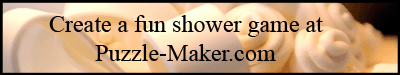Candy Aisle

Q  S  D  N  U  O  M  E  N  T  H  O  S  K  F  L

F  J  U  I  C  Y  F  R  U  I  T  P  T  N  C  Q

S  U  G  A  R  D  A  D  D  Y  R  W  B  U  A  G

M  I  L  K  Y  W  A  Y  C  E  I  L  I  H  K  S

W  P  C  R  U  N  C  H  E  X  R  O  G  G  O  E

E  B  U  T  T  E  R  F  I  N  G  E  R  I  O  C

X  T  F  M  U  G  E  L  B  B  U  B  E  B  Z  E

T  S  N  I  C  K  E  R  S  X  C  I  D  T  A  I

R  J  F  O  A  L  M  O  N  D  J  O  Y  C  B  P

A  M  A  E  R  C  N  S  E  I  K  O  O  C  R  S

T  H  R  E  E  M  U  S  K  E  T  E  E  R  S  E

M  &  M  S  T  U  N  H  C  E  E  B  K  R  S  S

S  E  S  S  I  K  S  Y  E  H  S  R  E  H  G  E

Y  Y  P  J  S  E  S  E  E  R  Y  B  M  Q  U  E

M  B  A  B  Y  R  U  T  H  L  O  O  K  A  H  R

K  D  K  K  J  W  R  I  G  G  L  E  Y  S  B  R

 Almond Joy Extra Reeses Baby Ruth Hersheys Reeses Pieces Bazooka Hugs Snickers Beech Nut Juicy Fruit Spree Big Hunk Kisses Sugar Daddy Big Red Look Three Musketeers Bubble Gum M&Ms Twix Butterfinger Menthos Wriggleys Cookies n Cream Milky Way Crunch Mounds